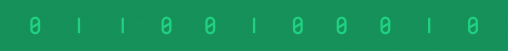#### Tag: pandasScatter plots are frequently used in data science and machine learning projects. In this pandas tutorial, I’ll show you two simple methods to plot one. Both solutions will…In this tutorial, you’ll learn how to run a Python script. And it’s quite essential. When working on data science projects, you’ll write Python code all the time……This is the third episode of my pandas tutorial series. In this one I’ll show you four data formatting methods that you might use a lot in data…Let’s continue with the pandas tutorial series. This is the second episode, where I’ll introduce aggregation (such as min, max, sum, count, etc.) and grouping. Both are very…Pandas is one of the most popular Python libraries for Data Science and Analytics. I like to say it’s the “SQL of Python.” Why? Because pandas helps you…